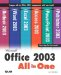# Index SYMBOL

 [SYMBOL] [A] [B] [C] [D] [E] [F] [G] [H] [I] [J] [K] [L] [M] [N] [O] [P] [Q] [R] [S] [T] [U] [V] [W] [X] [Y] [Z] ####### ( pound sign) messages (Excel)   \$ (dollar sign)        Excel cell referencing   " (quotation marks)        Excel functions   (equal to) symbol ()   (greater than or equal) to symbol   (greater than) symbol   (less than or equal to) symbol   (less than) symbol   * (multiplication operator)        Excel formulas   + (addition operator)        Excel formulas   - (subtraction operator)        Excel formulas   / (division operator)        Excel formulas   = (equal sign)        Excel formulas          Excel functions   [cw] copyright symbol   [en] en dashes   [md] em dashes   [tm] trademark symbol   ^ (exponentiation operator)        Excel formulasMicrosoft Office 2003 All-in-One
ISBN: B005HKSHB2
EAN: N/A
Year: 2002
Pages: 660
Authors: Joe Habraken

Similar book on Amazon